Liquid Studio Documentation
Data Mapping / Data Mapper Functions / By Category / Maths / Add
In This Topic
In This Topic

Maths
##### Icon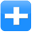##### Inputs
 Value 1 First value to add Value 2 Second value to add
##### Outputs
 Result Sum result of adding value 1 and value 2
##### Properties
 None -

##### Usage

The Data Mapper Maths Add function allows you to carry out addition on input values. You can use the Add function with input source data or in conjunction with other arithmetic functions in the Maths section. To apply the Maths Add function, use the following process:

Create a new Data Mapper file, dragging your XML data source and target into the editor area. For this example we are using payroll_source.xsd, an XML Schema Definition inferred from the following source:

Source XML
Copy Code
```<payroll>
<employee payroll_id="126">
<rate>9.45</rate>
<hours>35</hours>
<bonus>50.00</bonus>
<funding>350</funding>
</employee>
<employee payroll_id="129">
<rate>10.50</rate>
<hours>40</hours>
<bonus>150.00</bonus>
<funding>500</funding>
</employee>
<employee payroll_id="130">
<rate>8.90</rate>
<hours>35</hours>
<bonus>100.00</bonus>
<funding>400</funding>
</employee>
<employee payroll_id="133">
<rate>11.20</rate>
<hours>40</hours>
<bonus>200.00</bonus>
<funding>600</funding>
</employee>
</payroll>
```

For our target schema we will be using payroll_target.xsd, inferred from the following XML:

Target XML
Copy Code
```<wages>
<payee>
<ref>122</ref>
<result type="cost">500.00</result>
</payee>
<payee>
<ref>125</ref>
<result type="cost">475.50</result>
</payee>
<payee>
<ref>126</ref>
<result type="cost">600.20</result>
</payee>
</wages>
```

The data models payroll data for temporary staff within an organisation. The output is designed to capture some value calculated from the source, using the "type" attribute to indicate what the calculation result represents. Let's assume that we want to calculate the weekly cost of employing each staff member, including their bonus payment. To calculate this we will need to multiply the hourly rate by the number of hours worked, then add the bonus to the result. Here is the Data Mapper with Source and Target imported:Drag the Maths Add function from the Component Palette into the mapping area: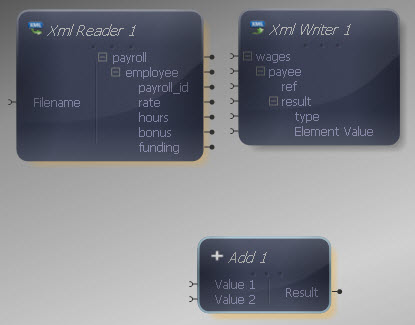The Add component has two inputs, representing the values to add. Both inputs and output of the Add function may be connected to items in the XML Reader/ Writer or other components in the Mapper.

Connect the first Add input to the "bonus" output in the XML Reader.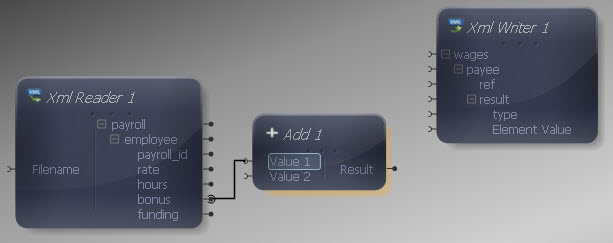For the second Add input, we need to carry out another calculation. Drag a Multiply component onto the Mapper, connecting its inputs to the "rate" and "hours" outputs in the XML Reader.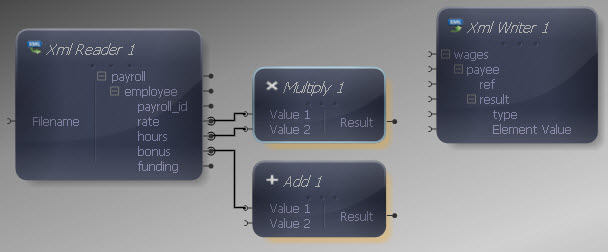Connect the output of the Multiply function to the remaining Add input.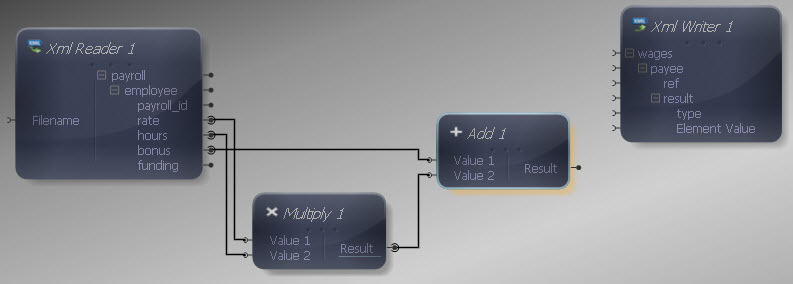Now connect the Add output to the "Element Value" input in the XML Writer, so that the addition result is written into the "result" element.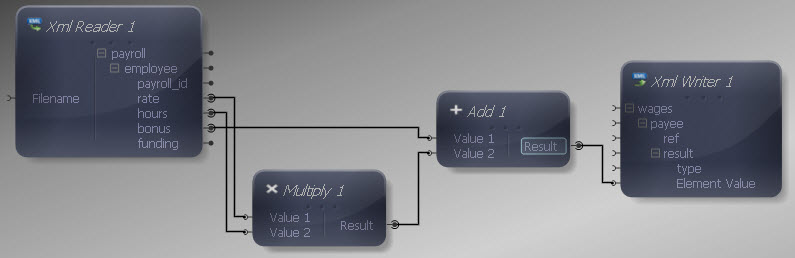Now we need to include the "type" attribute in the source, so add a Constant Value from the Data Type section. Right-click it and choose Show Properties. Choose a String Data Type and enter "cost" as the value.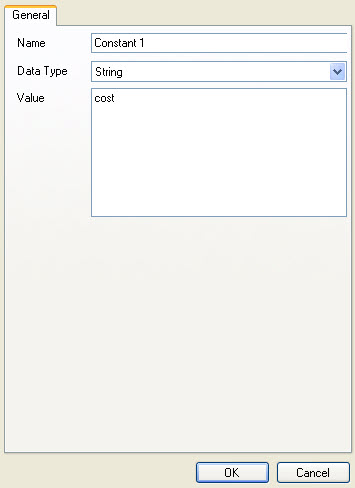Connect the Constant output to the "type" input in the XML Writer.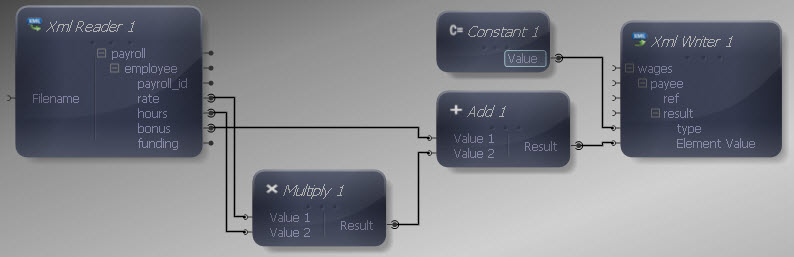Finally let's make the remaining input and output connections. We do not want to map all data items in the source, as we are only interested in certain values. Map "payroll" to "wages", "employee" to "payee" and "payroll_id" to "ref". We don't need to map anything to the "result" input as the element will be written out each time the Mapper maps the addition result to "Element Value".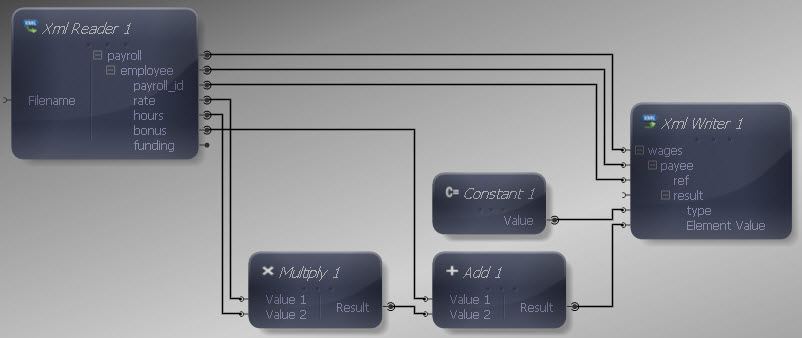We can now execute the transform by pressing Shift-F5 or the Execute button (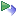). The transform is applied and the file we selected as output opens in the editor:

Output XML
Copy Code
```<wages>
<payee>
<ref>126</ref>
<result type="cost">380.75</result>
</payee>
<payee>
<ref>129</ref>
<result type="cost">570.00</result>
</payee>
<payee>
<ref>130</ref>
<result type="cost">411.50</result>
</payee>
<payee>
<ref>133</ref>
<result type="cost">648.00</result>
</payee>
</wages>
```

The output contains an indicator of the weekly cost for each employee, comprising their bonus plus rate multiplied by hours worked.

This is a scalar function so the standard rules apply if multiple values are presented to one or more inputs.